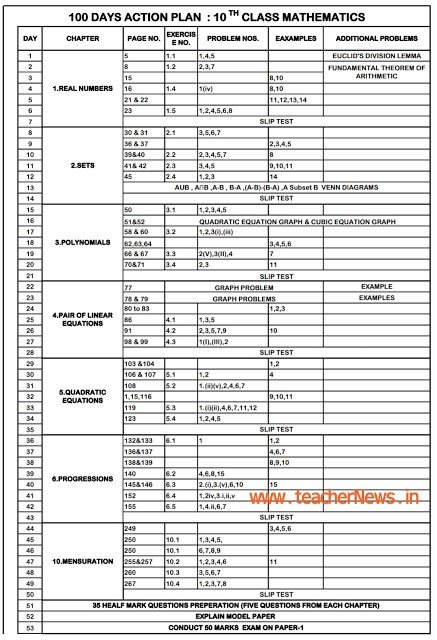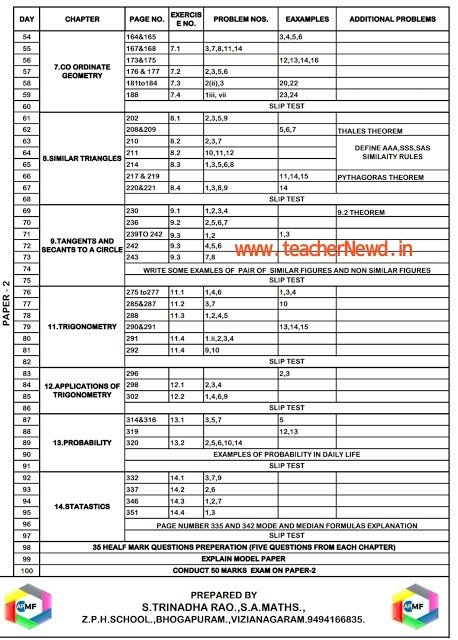# SSC Maths 100 days action plan May 2021 | Maths Paper 1, 2 action plan## SSC Maths 100 days action plan May 2021 | Maths Paper 1, 2 action plan

Contents

AP Maths Forum Members prepare 10th class 100 days action plan release. SSC Maths 100 days action plan May 2021 | Maths Paper 1, 2 action plan  download. Chapter wise Mathematics important problems and C & D Grade students imp questions given below. TS , AP SSC Maths Paper wise important questions march 2020.

### 10th Class Maths 100 days action plan May 2021 | Mathematics Paper 1, 2 action plan###10th Class Mathematics 100 Days Paper 1, 2 Action Plan Of May 2021

• Mathematics Real Numbers
• Mathematics Test 2
• Mathematics Test 3 Polynomials
• Maths Test 4 Leniar Equations
• Maths Test 5 Quardratic Equations
• Maths Test 6 Progressions
• Maths Test 7 Mensuration
• Maths Test 8 Coordinate Geomentary
• Maths Test 9 Similar Triangles
• Maths Test 10 Tangent And Secent To A Circle
• Maths Test 11 Trignomentry
• Maths Test 12 Probability
• Maths Test 13 Statistics